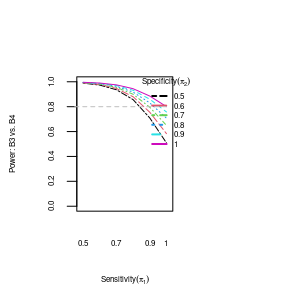# SMART: Single Sequential Treatment (SST)

The purpose of this developing this R package is to quantify and vidualize the misclassification effect on mean/variance of single sequential treatment (SST) and power comparasion of two SSTs in the setting of sequential multiple assigned randomized trial (SMART).

This vignette describes the use cases of SST for the smart package. Most of the trial settings and calculations are handled by the smartSST() function, where the arguments and settings follow the formula and terminology described in He et al (accepted).

This R-package was developed based on typical two-stage SMART design, where two initial treatments were denoted as treatment A1 and A2. After making the responder/non-responder designation based on an intermediate outcome, participants are then re-randomized into one of treatments B1 to B8 in treatment stage II. There are then four possible SSTs associated with A1 (A1B1 and A1B2 for responders; A1B3 and A1B4 for non-responders) and four for treatment A2 (A2B5 and A2B6 for responders; A2B7 and A2B8 for non-responders).

We denote the probability of participants being true responders as pG=p(G=1), where G is a binary indicator of the true responder status, and the probability of a participant being classified or observed as a responder as pR=p(R=1), where R is a binary indicator of the observed responder status. We define sensitivity, p(R=1|G=1), as the probability a participant is (correctly) classified as a responder conditional on their true status being a responder, and we define specificity, p(R=0|G=0), as the probability a participant is (correctly) classified as a non-responder conditional on their true status being a non-responder.

The following notation represents expected means and variances of the outcome (Y) at the end of the second stage. The expected mean outcome for responders is mu_BbGgRr=E(Y│B=b,G=g,R=r) with corresponding variance sigsq_BbGgRr, where B is defined as treatment group in stage II and subscript ‘b’ represents responders (b=s=1,2,5 or 6) or non-responders (b=t=3,4,7 or 8) to initial treatment. The subscript ‘s’ represents responders in stage II to initial treatment, while ‘t’ represents non-responders in stage II to initial treatment. Thus the expected mean outcome and variance for true responders classified as responders are mu_BsG1R1=E(Y│B=s,G=1,R=1) and sigsq_BsG1R1, and the expected mean outcome of true non-responders misclassified and receiving treatment ‘s’ is then mu_BsG0R1= E(Y│B=s,G=0,R=1) with variance sigsq_BsG0R1. Likewise, mu_BtG0R0=E(Y│B=t,G=0,R=0) represents the expected mean outcome of the true non-responders who were classified correctly (corresponding variance sigsq_BtG0R0), while mu_BtG1R0=E(Y│B=t,G=1,R=0) represents the expected mean outcome of true responders who were misclassified as non-responders (corresponding variance sigsq_BtG1R0). Then mu_Bs=E(Y|B=s) represents the expected mean outcome of the observed subjects receiving treatment ‘s’, with corresponding variance sigsq_Bs. To simplify formula notation, we drop the third subscript R because received treatment (B) already reflects the value of R (e.g., mu_BsG0R0=mu_BsG0). Further, the B and G in the subscripts are dropped (e.g., mu_BsG0=mu_(s,0), where ‘s’ represents responders in stage II to initial treatment, and ‘0’ represents G=0).

Although SMARTs can be designed for many different types of outcomes, this research considers only continuous outcomes at the end of the study (Stage II outcome).

# SST Calculations

We run smartSST for mean/variance/relative bias of SST of responders (eg. A1B1) and non-responders (eg. A1B3). Two different examples are presented, one for responder and the other for non-responder. The calculations needed for one run of smartSST are for one B-level and the G1,G0 pairing for that B-level.

In the first example, we run with B-level of 1 (responders), supposing the true responders (B1G1) who were classified to treatment B1 have a better response than true non-responders who were misclassified to treatment B1 (B1G0) with the means of (30, 20) for (G1, G0), respectively, with sample size of 252 and pG_A1 of 0.8. The variance between correctly classified and misclassified participants were assumed same variance of 16. The sensitivity and specificity ranges of 0.5 to 1 are for where the calculations are performed, but the range of sensitivity and specificity is (0,1]. ‘pran_Barm’ represents the probability that participants are assigned into corresponding Barm. For example, if pran_Barm=0.5, then the probability of being assigned to B1 group is 0.5. We show what is returned in the sst1 object, and the main calculations in the sstdat data.frame within the object.

Note that even though the formulae of mean/variance of SST didn’t contain the number of subject because the nsubject was canceled in the denominator and numerator, we still need to put any number that is >10 to generate the dummy variable which are used in the R program. However, the different nsubject will not change the results of the mean/variance calculation (need to double check whether it is true).

sst1 <- smartSST(mu_Barm = c(G1 = 30, G0 = 20), sigsq_Barm = c(G1 = 16, G0 = 16),
Barm = 1, sens = seq(0.5, 1, by = 0.1), spec = seq(0.5, 1, by = 0.1), nsubject = 252,
pG_A1 = 0.8, pran_A1 = 0.5, pran_Barm = 0.5)
names(sst1$sstdat)  "sens" "spec" "mu_Barm" "sigsq_Barm" "n_Barm"  sst1 smart SST object with B-level: 1 sens spec mu_Barm sigsq_Barm n_Barm 1 0.5 0.5 28.00000 32.00000 31.50 2 0.5 0.6 28.33333 29.88889 30.24 3 0.5 0.7 28.69565 27.34216 28.98 4 0.5 0.8 29.09091 24.26446 27.72 5 0.5 0.9 29.52381 20.53515 26.46 6 0.5 1.0 30.00000 16.00000 25.20 7 0.6 0.5 28.27586 30.26873 36.54 8 0.6 0.6 28.57143 28.24490 35.28 9 0.6 0.7 28.88889 25.87654 34.02 10 0.6 0.8 29.23077 23.10059 32.76 11 0.6 0.9 29.60000 19.84000 31.50 12 0.6 1.0 30.00000 16.00000 30.24 13 0.7 0.5 28.48485 28.85583 41.58 14 0.7 0.6 28.75000 26.93750 40.32 15 0.7 0.7 29.03226 24.74089 39.06 16 0.7 0.8 29.33333 22.22222 37.80 17 0.7 0.9 29.65517 19.32937 36.54 18 0.7 1.0 30.00000 16.00000 35.28 19 0.8 0.5 28.64865 27.68736 46.62 20 0.8 0.6 28.88889 25.87654 45.36 21 0.8 0.7 29.14286 23.83673 44.10 22 0.8 0.8 29.41176 21.53633 42.84 23 0.8 0.9 29.69697 18.93848 41.58 24 0.8 1.0 30.00000 16.00000 40.32 25 0.9 0.5 28.78049 26.70791 51.66 26 0.9 0.6 29.00000 25.00000 50.40 27 0.9 0.7 29.23077 23.10059 49.14 28 0.9 0.8 29.47368 20.98615 47.88 29 0.9 0.9 29.72973 18.62966 46.62 30 0.9 1.0 30.00000 16.00000 45.36 31 1.0 0.5 28.88889 25.87654 56.70 32 1.0 0.6 29.09091 24.26446 55.44 33 1.0 0.7 29.30233 22.48999 54.18 34 1.0 0.8 29.52381 20.53515 52.92 35 1.0 0.9 29.75610 18.37954 51.66 36 1.0 1.0 30.00000 16.00000 50.40 In the second example, we do similar calculations for non-responders (B-level of 3), but the formulae of mean/variances for responders and non-responders are different. We assumed that the true non-responders who were correctly classified to treatment B3 (B3G0) had worse treatment response than the true responders who were misclassfied to B3 (B3G1), with the means of (25, 20) for (G1, G0), respectively. Otherwise, the same settings as above. sst3 <- smartSST(mu_Barm = c(G1 = 25, G0 = 20), sigsq_Barm = c(G1 = 16, G0 = 16), Barm = 3, sens = seq(0.5, 1, by = 0.1), nsubject = 252, spec = seq(0.5, 1, by = 0.1), pG_A1 = 0.8) print(sst3, digits = 3) smart SST object with B-level: 3 sens spec mu_Barm sigsq_Barm n_Barm 1 0.5 0.5 24.0 20.0 31.50 2 0.5 0.6 23.8 20.4 32.76 3 0.5 0.7 23.7 20.8 34.02 4 0.5 0.8 23.6 21.1 35.28 5 0.5 0.9 23.4 21.4 36.54 6 0.5 1.0 23.3 21.6 37.80 7 0.6 0.5 23.8 20.5 26.46 8 0.6 0.6 23.6 21.0 27.72 9 0.6 0.7 23.5 21.3 28.98 10 0.6 0.8 23.3 21.6 30.24 11 0.6 0.9 23.2 21.8 31.50 12 0.6 1.0 23.1 21.9 32.76 13 0.7 0.5 23.5 21.2 21.42 14 0.7 0.6 23.3 21.6 22.68 15 0.7 0.7 23.2 21.8 23.94 16 0.7 0.8 23.0 22.0 25.20 17 0.7 0.9 22.9 22.1 26.46 18 0.7 1.0 22.7 22.2 27.72 19 0.8 0.5 23.1 21.9 16.38 20 0.8 0.6 22.9 22.1 17.64 21 0.8 0.7 22.7 22.2 18.90 22 0.8 0.8 22.5 22.2 20.16 23 0.8 0.9 22.4 22.2 21.42 24 0.8 1.0 22.2 22.2 22.68 25 0.9 0.5 22.2 22.2 11.34 26 0.9 0.6 22.0 22.0 12.60 27 0.9 0.7 21.8 21.8 13.86 28 0.9 0.8 21.7 21.6 15.12 29 0.9 0.9 21.5 21.3 16.38 30 0.9 1.0 21.4 21.1 17.64 31 1.0 0.5 20.0 16.0 6.30 32 1.0 0.6 20.0 16.0 7.56 33 1.0 0.7 20.0 16.0 8.82 34 1.0 0.8 20.0 16.0 10.08 35 1.0 0.9 20.0 16.0 11.34 36 1.0 1.0 20.0 16.0 12.60 ## SST Plots This is an example of plotting the mean of B1 from the first example for responders. Metric allows us to choose either “mean” or “variance”; xtype represents two choices in x-axis, specificity “spec” or sensitivity “sens”. When sens=spec=1, it represents the case without misclassification. “mar=c(4,4,4,6)” represents how much margins the figure left to the border (bottom, left, top, right). “legend.inset” represents the location of legend (horizontal, vertical). “cex.lab” represents the axis label size, “cex.axis” represents the axis annotation size. When “relativeBias=FALSE” (default), the plot shows raw data, which is the expected mean in this case. When “relativeBias=TRUE”, the plot shows relative bias, which is the relative bias of mean in this case. The function plot or plot.smartSST will show same results here. # plot(sst1, metric='mean', xtype='spec',cex.lab=1.5, cex.axis=1.2, # mar=c(5,5,2,9), legend.inset=c(-0.45,0.1)) plot.smartSST(sst1, metric = "mean", xtype = "spec", cex.lab = 1.5, cex.axis = 1.2, mar = c(5, 5, 2, 9), legend.inset = c(-0.45, 0.1), relativeBias = FALSE)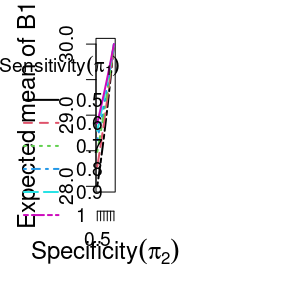This is an example of plotting the variance of B1 from the first example for responders. plot.smartSST(sst1, metric = "variance", xtype = "spec", cex.lab = 1.5, cex.axis = 1.2, mar = c(5, 5, 2, 9), legend.inset = c(-0.45, 0.1))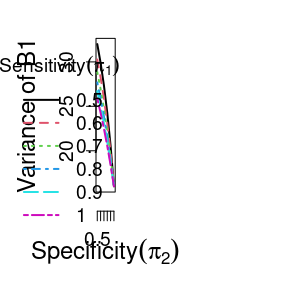This is an example of plotting the mean of B3 from the first example for non-responders. plot.smartSST(sst3, metric = "mean", xtype = "spec", ylim = c(-30, 10), mar = c(4, 4, 4, 6), relativeBias = TRUE)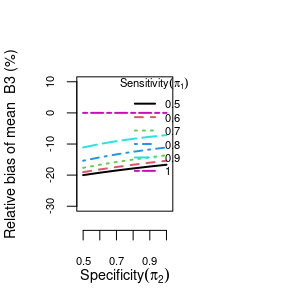This is an example of plotting the variance of B3 from the first example for non-responders. plot.smartSST(sst3, metric = "variance", xtype = "sens", mar = c(4, 4, 4, 6), cex.axis = 0.5, cex.lab = 0.5)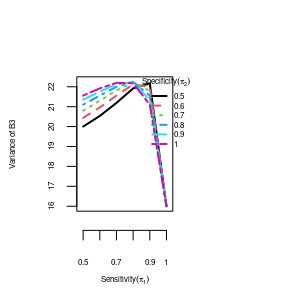## Relative Bias plots, separate for relative mean and relative variance (sigsq) This is an example of plotting the relative mean and relative variance. plot.smartSST(sst3, metric = "mean", xtype = "sens", cex.axis = 0.5, cex.lab = 0.5, mar = c(4, 4, 4, 6), relativeBias = TRUE)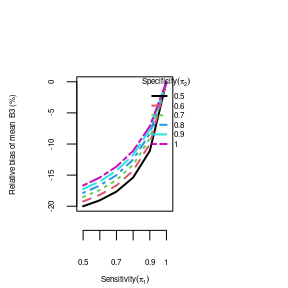# SST Power To evaluate the power comparison between two groups (eg.B1 versus B2, B3 versus B4), the calculated results from sst1 and sst2 are required. We will use two examples to show the misclassification effects on power based on targeted power of 0.8. The first example shows the power of comparison between responders (B1 vs. B2) with the condition that N=316, effect size=0.5 for true responders, and bigger effect size for true non-responders misclassified to responder group (0.9). ## need to add warnigns that power does not work if sens/spec are different sst1 <- smartSST(mu_Barm = c(G1 = 30, G0 = 20), sigsq_Barm = c(G1 = 100, G0 = 100), Barm = 1, sens = seq(0.5, 1, by = 0.1), nsubject = 316, spec = seq(0.5, 1, by = 0.1), pG_A1 = 0.8) sst2 <- smartSST(mu_Barm = c(G1 = 25, G0 = 11), sigsq_Barm = c(G1 = 100, G0 = 100), Barm = 2, sens = seq(0.5, 1, by = 0.1), nsubject = 316, spec = seq(0.5, 1, by = 0.1), pG_A1 = 0.8) powsst1vs2 <- powerSST(sst1, sst2, alpha = 0.05) ## access the full power data.frame/matrix powsst1vs2$powerdat  ## same as: print.powerSST(powsst1vs2)
   sens spec    mu_B1 sigsq_B1    mu_B2 sigsq_B2  z_Barm    pval   power
1   0.5  0.5 28.00000 116.0000 22.20000 131.3600 2.31772 0.02046 0.63973
2   0.5  0.6 28.33333 113.8889 22.66667 127.2222 2.24726 0.02462 0.61306
3   0.5  0.7 28.69565 111.3422 23.17391 122.2306 2.17800 0.02941 0.58630
4   0.5  0.8 29.09091 108.2645 23.72727 116.1984 2.11070 0.03480 0.55992
5   0.5  0.9 29.52381 104.5352 24.33333 108.8889 2.04656 0.04070 0.53452
6   0.5  1.0 30.00000 100.0000 25.00000 100.0000 1.98746 0.04687 0.51099
7   0.6  0.5 28.27586 114.2687 22.58621 127.9667 2.47454 0.01334 0.69657
8   0.6  0.6 28.57143 112.2449 23.00000 124.0000 2.41098 0.01591 0.67400
9   0.6  0.7 28.88889 109.8765 23.44444 119.3580 2.34868 0.01884 0.65125
10  0.6  0.8 29.23077 107.1006 23.92308 113.9172 2.28827 0.02212 0.62866
11  0.6  0.9 29.60000 103.8400 24.44000 107.5264 2.23064 0.02570 0.60668
12  0.6  1.0 30.00000 100.0000 25.00000 100.0000 2.17715 0.02947 0.58597
13  0.7  0.5 28.48485 112.8558 22.87879 125.1974 2.62365 0.00870 0.74655
14  0.7  0.6 28.75000 110.9375 23.25000 121.4375 2.56550 0.01030 0.72758
15  0.7  0.7 29.03226 108.7409 23.64516 117.1321 2.50860 0.01212 0.70837
16  0.7  0.8 29.33333 106.2222 24.06667 112.1956 2.45347 0.01415 0.68917
17  0.7  0.9 29.65517 103.3294 24.51724 106.5256 2.40080 0.01636 0.67033
18  0.7  1.0 30.00000 100.0000 25.00000 100.0000 2.35160 0.01869 0.65233
19  0.8  0.5 28.64865 111.6874 23.10811 122.9072 2.76581 0.00568 0.78983
20  0.8  0.6 28.88889 109.8765 23.44444 119.3580 2.71202 0.00669 0.77398
21  0.8  0.7 29.14286 107.8367 23.80000 115.3600 2.65945 0.00783 0.75787
22  0.8  0.8 29.41176 105.5363 24.17647 110.8512 2.60851 0.00909 0.74168
23  0.8  0.9 29.69697 102.9385 24.57576 105.7594 2.55976 0.01047 0.72567
24  0.8  1.0 30.00000 100.0000 25.00000 100.0000 2.51396 0.01194 0.71020
25  0.9  0.5 28.78049 110.7079 23.29268 120.9875 2.90175 0.00371 0.82684
26  0.9  0.6 29.00000 109.0000 23.60000 117.6400 2.85157 0.00435 0.81369
27  0.9  0.7 29.23077 107.1006 23.92308 113.9172 2.80255 0.00507 0.80026
28  0.9  0.8 29.47368 104.9861 24.26316 109.7729 2.75503 0.00587 0.78670
29  0.9  0.9 29.72973 102.6297 24.62162 105.1541 2.70947 0.00674 0.77321
30  0.9  1.0 30.00000 100.0000 25.00000 100.0000 2.66646 0.00767 0.76005
31  1.0  0.5 28.88889 109.8765 23.44444 119.3580 3.03213 0.00243 0.85817
32  1.0  0.6 29.09091 108.2645 23.72727 116.1984 2.98498 0.00284 0.84732
33  1.0  0.7 29.30233 106.4900 24.02326 112.7204 2.93893 0.00329 0.83619
34  1.0  0.8 29.52381 104.5352 24.33333 108.8889 2.89427 0.00380 0.82492
35  1.0  0.9 29.75610 102.3795 24.65854 104.6639 2.85136 0.00435 0.81363
36  1.0  1.0 30.00000 100.0000 25.00000 100.0000 2.81069 0.00494 0.80253
plot.powerSST(powsst1vs2, xtype = "sens", hline = 0.8, ylim = c(0, 1), mar = c(4,
4, 4, 6), cex.axis = 0.5, cex.lab = 0.5)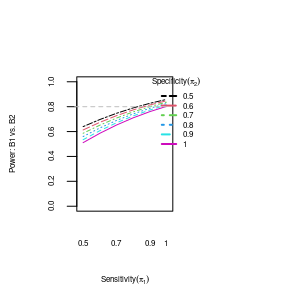plot.powerSST(powsst1vs2, xtype = "sens", alpha = 0.1, ylim = c(0.5, 1), hline = 0.9,
mar = c(4, 4, 4, 6), cex.axis = 0.5, cex.lab = 0.5)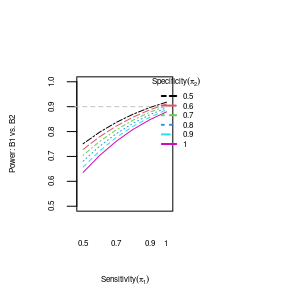The second example shows the power of comparison between non-responders (B3 vs. B4) with the condition that has N=1256, the effect size=0.5 for non-responders, and same effect size for SST true responders misclassified to non-responder group (0.5).

## need to add warnigns that power does not work if sens/spec are different
sst3 <- smartSST(mu_Barm = c(G1 = 35, G0 = 28), sigsq_Barm = c(G1 = 100, G0 = 100),
Barm = 3, sens = seq(0.5, 1, by = 0.1), nsubject = 1256, spec = seq(0.5, 1, by = 0.1),
pG_A1 = 0.8)
sst4 <- smartSST(mu_Barm = c(G1 = 30, G0 = 23), sigsq_Barm = c(G1 = 100, G0 = 100),
Barm = 4, sens = seq(0.5, 1, by = 0.1), nsubject = 1256, spec = seq(0.5, 1, by = 0.1),
pG_A1 = 0.8)

powsst3vs4 <- powerSST(sst3, sst4, alpha = 0.05)
## access the full power data.frame/matrix
powsst3vs4\$powerdat  ## same as: print.powerSST(powsst3vs4)
   sens spec    mu_B3 sigsq_B3    mu_B4 sigsq_B4  z_Barm    pval   power
1   0.5  0.5 33.60000 107.8400 28.60000 107.8400 4.26594 0.00002 0.98944
2   0.5  0.6 33.38462 108.6982 28.38462 108.6982 4.33322 0.00001 0.99118
3   0.5  0.7 33.18519 109.4102 28.18519 109.4102 4.40137 0.00001 0.99268
4   0.5  0.8 33.00000 110.0000 28.00000 110.0000 4.47010 0.00001 0.99397
5   0.5  0.9 32.82759 110.4875 27.82759 110.4875 4.53918 0.00001 0.99505
6   0.5  1.0 32.66667 110.8889 27.66667 110.8889 4.60841 0.00000 0.99596
7   0.6  0.5 33.33333 108.8889 28.33333 108.8889 3.89092 0.00010 0.97325
8   0.6  0.6 33.09091 109.7190 28.09091 109.7190 3.96739 0.00007 0.97765
9   0.6  0.7 32.86957 110.3743 27.86957 110.3743 4.04450 0.00005 0.98144
10  0.6  0.8 32.66667 110.8889 27.66667 110.8889 4.12189 0.00004 0.98469
11  0.6  0.9 32.48000 111.2896 27.48000 111.2896 4.19931 0.00003 0.98743
12  0.6  1.0 32.30769 111.5976 27.30769 111.5976 4.27655 0.00002 0.98974
13  0.7  0.5 32.94118 110.1730 27.94118 110.1730 3.48034 0.00050 0.93579
14  0.7  0.6 32.66667 110.8889 27.66667 110.8889 3.56966 0.00036 0.94626
15  0.7  0.7 32.42105 111.4017 27.42105 111.4017 3.65903 0.00025 0.95534
16  0.7  0.8 32.20000 111.7600 27.20000 111.7600 3.74806 0.00018 0.96312
17  0.7  0.9 32.00000 112.0000 27.00000 112.0000 3.83650 0.00012 0.96971
18  0.7  1.0 31.81818 112.1488 26.81818 112.1488 3.92418 0.00009 0.97525
19  0.8  0.5 32.30769 111.5976 27.30769 111.5976 3.02398 0.00249 0.85633
20  0.8  0.6 32.00000 112.0000 27.00000 112.0000 3.13249 0.00173 0.87950
21  0.8  0.7 31.73333 112.1956 26.73333 112.1956 3.23961 0.00120 0.89966
22  0.8  0.8 31.50000 112.2500 26.50000 112.2500 3.34504 0.00082 0.91698
23  0.8  0.9 31.29412 112.2076 26.29412 112.2076 3.44864 0.00056 0.93171
24  0.8  1.0 31.11111 112.0988 26.11111 112.0988 3.55035 0.00038 0.94412
25  0.9  0.5 31.11111 112.0988 26.11111 112.0988 2.51047 0.01206 0.70901
26  0.9  0.6 30.80000 111.7600 25.80000 111.7600 2.65028 0.00804 0.75499
27  0.9  0.7 30.54545 111.3388 25.54545 111.3388 2.78489 0.00535 0.79528
28  0.9  0.8 30.33333 110.8889 25.33333 110.8889 2.91462 0.00356 0.83011
29  0.9  0.9 30.15385 110.4379 25.15385 110.4379 3.03982 0.00237 0.85989
30  0.9  1.0 30.00000 110.0000 25.00000 110.0000 3.16084 0.00157 0.88509
31  1.0  0.5 28.00000 100.0000 23.00000 100.0000 1.98116 0.04757 0.50848
32  1.0  0.6 28.00000 100.0000 23.00000 100.0000 2.17025 0.02999 0.58328
33  1.0  0.7 28.00000 100.0000 23.00000 100.0000 2.34414 0.01907 0.64957
34  1.0  0.8 28.00000 100.0000 23.00000 100.0000 2.50599 0.01221 0.70747
35  1.0  0.9 28.00000 100.0000 23.00000 100.0000 2.65801 0.00786 0.75742
36  1.0  1.0 28.00000 100.0000 23.00000 100.0000 2.80179 0.00508 0.80005
plot.powerSST(powsst3vs4, xtype = "sens", hline = 0.8, ylim = c(0, 1), cex.axis = 0.5,
cex.lab = 0.5, mar = c(4, 4, 4, 6), legend.inset = c(-0.2, 0))